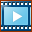﻿ Step 5.1: Function-Blocks, Connectors and Wires

# Step 5.1: Function-Blocks, Connectors and Wires

## Function-Blocks, Connectors and Wires

#### Function-Blocks [FBs], Connectors and WiresThe icon of each Function-Blocks [FB] should help you recognize its 'function'.

In the image, there are two FBs: the Linear-Motion FB [clock face!] and a Motion-Dimension FB

The small triangular symbolsare 'out-from' and 'in-to' each FB.

 • The output-connectoris to the right of a FB
 • There input-connectoris to the left of a FB

Motion-values 'flow' from FB to FB, along wiresfrom an output-connectorto an input-connector.

#### To connect FBsDrag Wires from Output-Connector to Input-Connectors

In the image to the left, there are four Function-Blocks [FBs] with wires that connect them.

The motion-values flow from the output-connector of a Linear-Motion FB, and along each wire and through each of the other three FBs to specify the angle of a rotating-part with a Motion-Dimension FB

To connect wires between two Function-Blocks:

 1 Move your mouse-pointer above the output-connectorof a FB so that the output-connector becomes red ...
 2 ...mouse-button down...
 3 ...and drag a wirefrom the output-connector to the input-connector of a different FB...so that it becomes red...
 4 ...mouse-button-up.

Now, a wire is between the output-connector and the input-connector of two FBs.

 5 Do 1 to 4 again and again to add more wires.Video to show how to connect wires between Function-Block connectors.

There is an equation associated with each type of Function-Block. The equation has parameters that you can edit.

The Input and Output of the Function-Block

When the Master-Machine-Angle changes, a motion-value at the input-connector of a Function-Block instantly becomes a different motion-value at its output-connector.

The motion-values also flow instantly along wires between Function-Blocks

The last function-block type usually specifies the position of a Part. Thus, the position of a Part updates instantly when the Master Machine Angle changes.Example: of the 'flow' of motion-values along the wires of four FBs:---1 Linear-Motion FBThe [default] motion-values at the output-connector of this Linear-Motion FB are the same as the Master-Machine-Angle [ 0 → 360]

Therefore, as you cycle the machine, the output from the 'first FB' changes at the same rate as the Master-Machine-Angle.

 2 Motion FBThe Motion FB makes a link, similar to a hyperlink, with a motion name in MotionDesigner.

The motion-values at its input-connector relate to the X-axis values of a motion. The motion-values at its output-connector are the Y-axis values of the same motion. You edit the Motion FB to 'hyper-link' a different motion name, in a Motion tab in MotionDesigner.

 3 Gearing FBThe Gearing FB has three parameters to multiply motion-values at its input connector by a fixed number, add or subtract a number.

If the input is 0 - 360, and the Gearing Ratio parameter is 2, and you add 40 to the output [after you multiply by 2], then its output will change from 40 to 760.

 4 Motion-Dimension FBThe Motion-Dimension FB uses the motion-values at its input-connector to control the motion of a Motion-Part. The Motion-Part in this case is a 'Rocker'.

It is possible to offset the initial position of the Rocker . You must edit Base-Value parameter with the Motion-Dimension FB.

Thus, if the Base-Value is '20', the Rocker moves from 60º to 780º within the 0 – 360 cycle of the Master-Machine-Angle.

#### The Kinematic Function-Blocks

Motion Providers: 'to provide' motion-values.• Linear-Motion FB
 • One output-connector• Measurement FB [Point to Line [Linear]; Line to Line[Angle]; 3 Points [Angle]]
 • One output-connector• Measurement FB [Point to Point [Linear]]
 • Two output-connectors• Point-Data FB
 • Three output-connectors

Motion Processors: 'to process', or 'to manipulate' the motion-data.• Motion FB
 • One input-connector and one output-connector• Gearing FB
 • One input-connector and one output-connector

Motion-Parts: 'to transfer' the angular or positional motion-values to a Motion-Part with a Motion-Dimension.• Motion-Dimension FB – Rocker
 • One input-connector and one output-connector• Motion-Dimension FB – Slider
 • One input-connector and one output-connector

Motion-Paths: 'to transfer' motion-values to a Motion-Point to define its position along a sketch-loop.• Motion-Path FB
 • One input-connector

Tutorial and Reference Help Files for MechDesigner and MotionDesigner 13.2 + © Machine, Mechanism, Motion and Cam Design Software by PSMotion Ltd